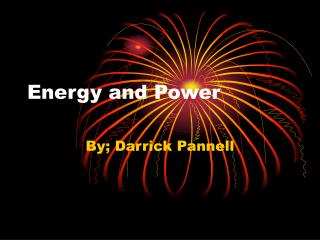# Energy and Power - PowerPoint PPT PresentationDownload PresentationEnergy and Power

Energy and PowerDownload Presentation## Energy and Power

- - - - - - - - - - - - - - - - - - - - - - - - - - - E N D - - - - - - - - - - - - - - - - - - - - - - - - - - -
##### Presentation Transcript

1. Energy and Power By; Darrick Pannell

2. What is Energy? • Energy is the power to change things. It is the ability to do work.

3. Kinetic Energy • The kinetic energy of an object is the energy it possesses because of its motion.

4. Potential Energy • Potential energy is the stored energy of position possessed by an object.

5. Mechanical Energy • The energy acquired by the objects upon which work is done is known as mechanical energy.

6. Thermal Energy • Thermal energy is the kinetic energy of the movement of atoms and molecules.

7. Electrical • Electrical energy is the scientific form of electricity, and refers to the flow of power or the flow of charges along a conductor to create energy.

8. Energy of Conversion • The process of changing one form of energy into another , such as nuclear energy into heat or solar energy into electrical energy.

9. Law of Conservation of Energy • The law of conservation of energy states that energy may neither be created nor destroyed.

10. Resources • 1. book • 2. www.brainpop.com • 3.www.kids.esdb.bg/index.html